### The Gradient of a Straight Line Graph

The gradient (slope) of the a straight line is: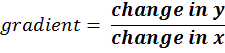(1)   y = ax + c

(2)   ax + by = c

#### Example 1.   Find the gradient of the graph y = x

(a) From example 1 of Straight Line Graphs is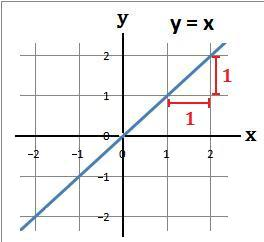(b) Work out the gradient. From the graph every time x increases by 1, y increases by 1 so

#### Example 2.   Find the gradient of the graph y = x + 2

(a) From example 2 of Straight Line Graphs is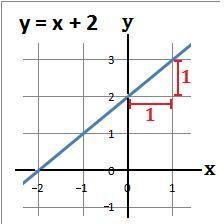(b) Work out the gradient. From the graph every time x increases by 1, y increases by 1 so

#### Example 3.   Find the gradient of the graph y = 2x + 3

(a) From example 3 of Straight Line Graphs is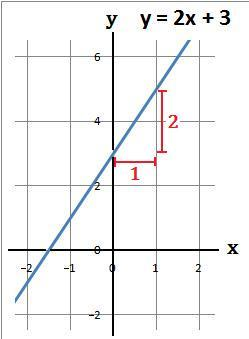(b) Work out the gradient. From the graph every time x increases by 1, y increases by 2 so

#### Example 4.   Find the gradient of the graph 4y − 4x = 3

(a) From example 4 of Straight Line Graphs is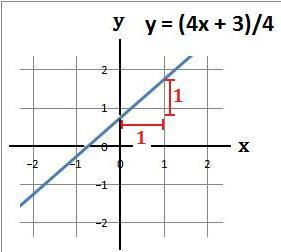(b) Work out the gradient. From the graph every time x increases by 1, y increases by 1 soto: